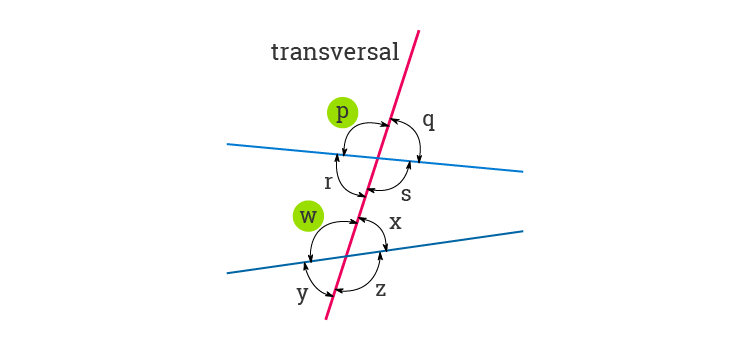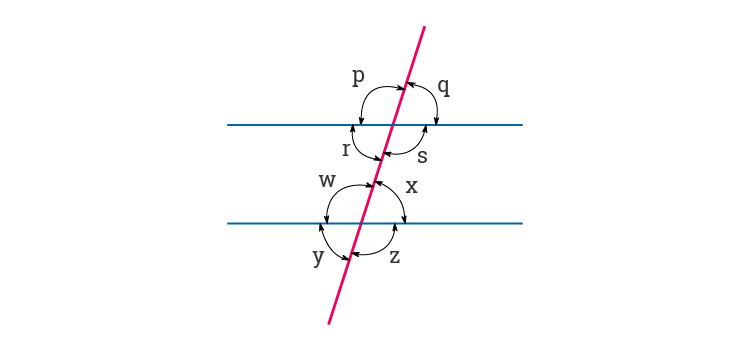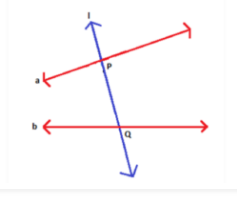Definition: Corresponding angles are the angles which are formed in matching corners or corresponding corners with the transversal when two parallel lines are intersected by any other line (i.e. the transversal). For example, in the below-given figure, angle p and angle w are the corresponding angles.Corresponding Angles

## Corresponding Angles Examples and Types

Examples of the corresponding angle are any angles which are formed on the opposite side of the transversal. Now, it should be noted that the transversal can intersect either two parallel line or two non-parallel lines. Thus, corresponding angles can be of two types:

• Corresponding angles formed by parallel lines and transversals
• Corresponding angles formed by non-parallel lines and transversals

In Maths, you must have learned about different types of lines and angles. Here we will discuss only corresponding angles formed by the intersection of two lines by a transversal. The two lines could be parallel or non-parallel. So, let us learn corresponding angles for both the cases.

### Corresponding Angles Formed by Parallel Lines and Transversals

If a line or a transversal crosses any two given parallel lines, then the corresponding angles formed have equal measure. In the given figure, you can see, the two parallel lines are intersected by a transversal, which forms eight angles with the transversal. So, the angles formed by the first line with transversal have equal corresponding angles formed by the second line with the transversal.Corresponding Angles Formed by Parallel Lines and Transversals

All corresponding angle pairs in the figure:

• ∠p and ∠w
• ∠q and ∠x
• ∠r and ∠y
• ∠s and ∠z

Note: The corresponding angles formed by two parallel lines are always equal. So,

∠p = ∠w

∠q= ∠x

∠r = ∠y

And ∠s = ∠z

You should also note down, apart from corresponding angles, there are other angles formed when a transversal intersects two parallel lines. All the angles formed in the figure are:

Angle Type Definition Angle Relationships
Corresponding Angles Angles formed at the same relative position at each intersection. ∠p = ∠w, ∠q= ∠x, ∠r = ∠y, and ∠s = ∠z
Vertically Opposite angles The angles formed opposite to each other by a transversal. ∠p = ∠s, ∠q = ∠r, ∠w = ∠ z and ∠x = ∠y
Alternate Interior Angles The angles formed at the interior side or inside the two parallel lines with a transversal. ∠r = ∠x and ∠s = ∠w
Alternate Exterior Angles The angles formed at the outside or exterior side of the two parallel lines with a transversal. ∠p = ∠z and ∠q = ∠y
Consecutive Interior Angles/Co-interior Angles The angles formed inside the two parallel lines but one side of the transversal is the consecutive interior angles. The angles are supplementary to each other, that means the sum of these two angles is 180°. ∠r + ∠w = 180°
and ∠s + ∠x = 180°

### Corresponding Angles Formed by Non-Parallel Lines and Transversals

For non-parallel lines, if a transversal intersects them, then the corresponding angles formed doesn’t have any relation with each other. They are not equal as in the case of parallel lines but all are corresponding to each other.Corresponding Angles Formed by Non-Parallel Lines and Transversals

In the same, there is no relationship between the interior angles, exterior angles, vertically opposite angles and consecutive angles, in the case of the intersection of two non-parallel lines by a transversal.

## Corresponding Angles Postulate

The corresponding angle postulate states that the corresponding angles are congruent if the transversal intersects two parallel lines. In other words, if a transversal intersects two parallel lines, the corresponding angles will be always equal.

### Corresponding Angles in a Triangle

Corresponding angles in a triangle are those angles which are contained by a congruent pair of sides of two similar (or congruent) triangles. Corresponding angles in a triangle have the same measure.

## Corresponding Angles Theorem

The statement of the corresponding angles theorem states that “when a line intersects 2 parallel lines, then the corresponding angles in the 2 regions of intersection are congruent”.

## Converse Of Corresponding Angles Theorem

The converse of the statement that “If the corresponding angles in the two regions of intersections are congruent, then the lines are parallel in nature”.

What happens when a transversal intersects the 2 parallel lines and the set of corresponding angles are the same?

The 2 lines when intersected by a transversal are parallel. It is the converse of the corresponding angle theorem.

## Important Points On Corresponding Angles

• In the case of two parallel lines intersected by a third one, the angles that employ the same relative position at every intersection are termed corresponding angles to one another.
• The corresponding angles are congruent in nature to one another.
• In the case where the corresponding angles in the 2 regions of intersections are congruent, then the two lines are parallel in nature.

## Video Lesson on Types of Angles## Corresponding Angles Examples

Example 1: The two corresponding angles are given to be 9x + 10 and 55. What is the value of x?

The two given corresponding angles are congruent.

9x + 10 = 55

9x = 55 – 10

9x = 45

x = 5

Example 2: The values of two corresponding angles are given to be 7y – 12 and 5y + 6. What is the magnitude of each corresponding angle?

The values of two corresponding angles are given to be 7y – 12 and 5y + 6.

To find the value of y.

The two given corresponding angles are congruent.

7y – 12 = 5y + 6

7y – 5y = 12 + 6

2y = 18

y = 9

The magnitude of each corresponding angle,

5y + 6 = 5 (9) + 6 = 51

7y – 12 = 7 * (9) – 12 = 51

Example 3: The values of two corresponding angles ∠1 = 3x + 1 and ∠5 = 4x – 3. Solve for the value of x.

As they are corresponding angles and the lines are said to be parallel in nature, then they should be congruent.

Equate the given expressions ∠1 = 3x + 1 and ∠5 = 4x – 3 and find the value of x.

3x + 1 = 4x – 3

1 + 3 = 4x – 3x

4 = x

Hence the value of x is 4.

Example 4: The values of two corresponding angles ∠2 = 5x + 2 and ∠6 = 3x + 10. Solve for the value of x.

As they are corresponding angles and the lines are said to be parallel in nature, then they should be congruent.

Equate the given expressions ∠2 = 5x + 2 and ∠6 = 3x + 10 and find the value of x.

5x + 2 = 3x + 10

5x – 3x = 10 – 2

2x = 8

x = 8 / 2

x = 4

Hence the value of x is 4.

Example 5: The values of two corresponding angles ∠7 = 5x + 5 and ∠3 = 8x – 10. Solve for the value of x.

As they are corresponding angles and the lines are said to be parallel in nature, then they should be congruent.

Equate the given expressions ∠7 = 5x + 5 and ∠3 = 8x – 10 and find the value of x.

5x + 5 = 8x – 10

5 + 10 = 8x – 5x

15 = 3x

x = 15 / 3

x = 5

Hence the value of x is 5.

Subscribe to BYJU’S to get all the learning materials for Maths and Science subject. Also, download its app to get personalised videos content.

Q1

### Can Corresponding Angles be Supplementary?

Corresponding angles can be supplementary if the transversal intersects two parallel lines perpendicularly (i.e. at 90 degrees). In such case, each of the corresponding angles will be 90 degrees and their sum will add up to 180 degrees (i.e. supplementary).

Q2

### Are all Corresponding Angles Equal?

No, all corresponding angles are not equal. The corresponding angles which are formed when a transversal intersects two parallel lines are equal.

Q3

### What is the Angle Rule for Corresponding Angles?

The angle rule of corresponding angles or the corresponding angles postulates states that the corresponding angles are equal if a transversal cuts two parallel lines.

Q4

### What are the Types of Corresponding Angles Based on their Sum?

Based on their sum, corresponding angles can be:

• Supplementary Corresponding Angles (if their sum is 180 degree)
• Complementary Corresponding angles (if their sum is 90 degrees)